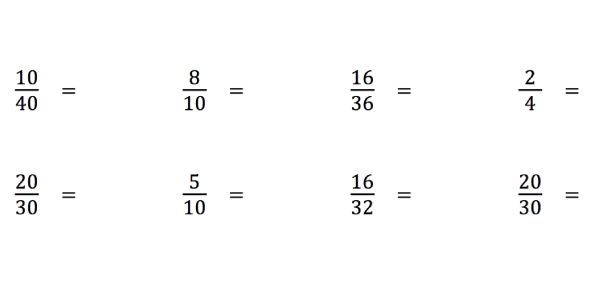# Comparing And Simplifying Fractions: Quiz

Approved & Edited by ProProfs Editorial Team
At ProProfs Quizzes, our dedicated in-house team of experts takes pride in their work. With a sharp eye for detail, they meticulously review each quiz. This ensures that every quiz, taken by over 100 million users, meets our standards of accuracy, clarity, and engagement.
| Written by Mathdancer
M
Mathdancer
Community Contributor
Quizzes Created: 3 | Total Attempts: 1,620
Questions: 10 | Attempts: 1,502SettingsMath is quite an interesting subject to study, and if you have a proper understanding of it, you will be all set for the classes you will have later. Do you have a difficult time when it comes to fractions? This is a short quiz over TURBO MATH Fractions Lesson 1-4 comparing, equivalent, simplifying, and adding fractions. Give it a try!

• 1.

### Compare: 3/4                        5/6

Explanation
The fraction 3/4 is smaller than the fraction 5/6. This can be determined by comparing the numerators (3 and 5) and denominators (4 and 6) of the fractions. In this case, the numerator of the first fraction is smaller than the numerator of the second fraction, while the denominators are the same. Therefore, the first fraction is smaller than the second fraction.

Rate this question:

• 2.

### Compare 3/4              3/8

Explanation
The fraction 3/4 is greater than the fraction 3/8. This is because the numerator (3) is the same in both fractions, but the denominator (4) is greater than the denominator (8). Since the denominator represents the number of equal parts the whole is divided into, a larger denominator means each part is smaller. Therefore, the fraction with the larger denominator has smaller parts and is greater in value.

Rate this question:

• 3.

### Joe has 2/5 of an apple pie.  Susie has 1/3 of a cherry pie.  Who has the greatest amount?

Explanation
Joe has the greatest amount because 2/5 is a larger fraction than 1/3.

Rate this question:

• 4.

### Which fraction is equivalent to 5/6?

• A.

5/12

• B.

10/6

• C.

10/12

C. 10/12
Explanation
The fraction 10/12 is equivalent to 5/6 because both fractions represent the same value. To find an equivalent fraction, we can multiply or divide both the numerator and the denominator by the same number. In this case, we can multiply 5 by 2 and 6 by 2 to get 10/12. Therefore, 10/12 is equivalent to 5/6.

Rate this question:

• 5.

### Find the fraction equivalent to 2/3

• A.

6/9

• B.

14/21

• C.

Both

C. Both
Explanation
Both 6/9 and 14/21 are equivalent fractions to 2/3. To find equivalent fractions, we can multiply or divide both the numerator and denominator by the same non-zero number. In this case, we can multiply 2/3 by 2 to get 4/6, and then multiply again by 3 to get 6/9. Similarly, we can multiply 2/3 by 7 to get 14/21. Therefore, both 6/9 and 14/21 are equivalent fractions to 2/3.

Rate this question:

• 6.

### Simplify 7/8 =

7/8
Explanation
The given equation simplifies to 7/8.

Rate this question:

• 7.

### Simplify There are 24 students in Mrs. Nantz's class.  Six of the students walk to school every day.  What fraction of the students do not walk to school;?write in simplest form.

3/4
Explanation
To find the fraction of students who do not walk to school, we need to subtract the fraction of students who walk to school from 1 (since all the students fall into either of the two categories - walking or not walking). Since 6 out of 24 students walk to school, the fraction of students who walk is 6/24. Subtracting this from 1, we get 18/24. Simplifying this fraction, we get 3/4. Therefore, 3/4 is the fraction of students who do not walk to school.

Rate this question:

• 8.

3/4
• 9.

### Add & Simplify 7/9 + 2/9 =

1
Explanation
The given question asks to add the fractions 7/9 and 2/9. Since the denominators of both fractions are the same, we can simply add the numerators to get the result. Therefore, 7/9 + 2/9 equals 9/9, which can be simplified to 1.

Rate this question:

• 10.

### At Mayfest Cookoff, 6/12 of the dishes were cooked by boys, girls cooked 5/12, and girls cooked 1/12. What fraction of the dishes were cooked by kids?Back to top